# Rules for exponents

## Summary and examples

Exponents are heavily used in mathematics and in almost all applications. Therefore it is very important to know and understand the rules for exponents as given below

1.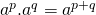2.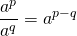3.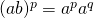4.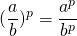5.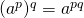6.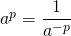7.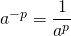8.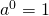9.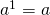10.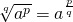Some remarks have to be made.
The operations 6 and 7 have to be understood as follows: a power function in the numerator of a fraction may be moved to the denominator if the sign of the exponent is changed. This also applies to a power function in the denominator of a fraction when it is moved to the numerator.
For some reason some students believe that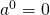, but this is wrong. The correct answer can be deduced by using rule 2 if you take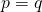.
Below a number of examples are given to find out how well you can apply rules for exponents. More exercises can be found in the Assignments.

##### Example 1

Simplify: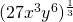This expression can be written as: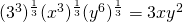##### Example 2

Simplify: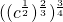This expression can be written as: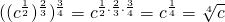##### Example 3

Simplify: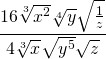This expression can be written as: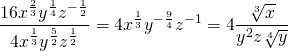##### Example 4

Simplify: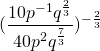This expression can be written as: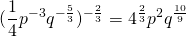0